• c语言实现归并排序
2021-05-19 12:59:19

下面是编程之家 jb51.cc 通过网络收集整理的代码片段。

编程之家小编现在分享给大家，也给大家做个参考。

// Mix two sorted tables in one and split the result into these two tables.

int *Mix(int *tab1,int *tab2,int count1,int count2)

{

int i,i1,i2;

i = i1 = i2 = 0;

int * temp = (int *)malloc(sizeof(int)*(count1+count2));

while((i1

{

while((i1

{

*(temp+i++) = *(tab1+i1);

i1++;

}

if (i1

{

while((i2

{

*(temp+i++) = *(tab2+i2);

i2++;

}

}

}

memcpy(temp+i,tab1+i1,(count1-i1)*sizeof(int));

memcpy(tab1,temp,count1*sizeof(int));

memcpy(temp+i,tab2+i2,(count2-i2)*sizeof(int));

memcpy(tab2,temp+count1,count2*sizeof(int));

// These two lines can be:

// memcpy(tab2,i2*sizeof(int));

free(temp);

}

// MergeSort a table of integer of size count.

// Never tested.

void MergeSort(int *tab,int count)

{

if (count==1) return;

MergeSort(tab,count/2);

MergeSort(tab+count/2,(count+1)/2);

Mix(tab,tab+count/2,count/2,(count+1)/2);

}

以上是编程之家(jb51.cc)为你收集整理的全部代码内容，希望文章能够帮你解决所遇到的程序开发问题。

如果觉得编程之家网站内容还不错，欢迎将编程之家网站推荐给程序员好友。

总结

以上是编程之家为你收集整理的C语言实现归并排序算法代码全部内容，希望文章能够帮你解决C语言实现归并排序算法代码所遇到的程序开发问题。

如果觉得编程之家网站内容还不错，欢迎将编程之家网站推荐给程序员好友。

本图文内容来源于网友网络收集整理提供，作为学习参考使用，版权属于原作者。

小编个人微信号 jb51ccc

喜欢与人分享编程技术与工作经验，欢迎加入编程之家官方交流群！

更多相关内容
• ## C语言实现归并排序

千次阅读 2022-04-07 12:46:43
归并排序每次递归的动作： 将给定数组以中点为界，分为左右两部分，且左右两边的数字分别有序。（至于如何将它做到分别有序，则需使用递归实现） 使用两个指针 L(初始值为左边界) 和 R(初始值为中点右侧的第一个...

### 思路：

创建一个动态空间(*p)作为排序辅助数组，其大小为(right - left + 1) * sizeof(int)。

归并排序每次递归的动作：

1. 将给定数组以中点为界，分为左右两部分，且左右两边的数字分别有序。（至于如何将它做到分别有序，则需使用递归实现）
2. 使用两个指针 L(初始值为左边界) 和 R(初始值为中点右侧的第一个数字 mid + 1) ，分别指向左右两边的左节点，比较指针指向的两个数字；
3. 如果左侧的数字小于等于右侧的数字，则将左侧的数字拷贝进入动态空间p，然后L++;
4. 如果左侧数字大于右侧数字，则将右侧数字拷贝进入动态空间p，然后R++;
5. 当左侧或者右侧数据拷贝完毕，即 !L <= mid 或者 !R <= right ，则停止比较。
6. 拷贝左侧或者右侧剩下的数字到p。
7. 将p的内容拷贝至原数组。

图解：

1. 分边和指针位置：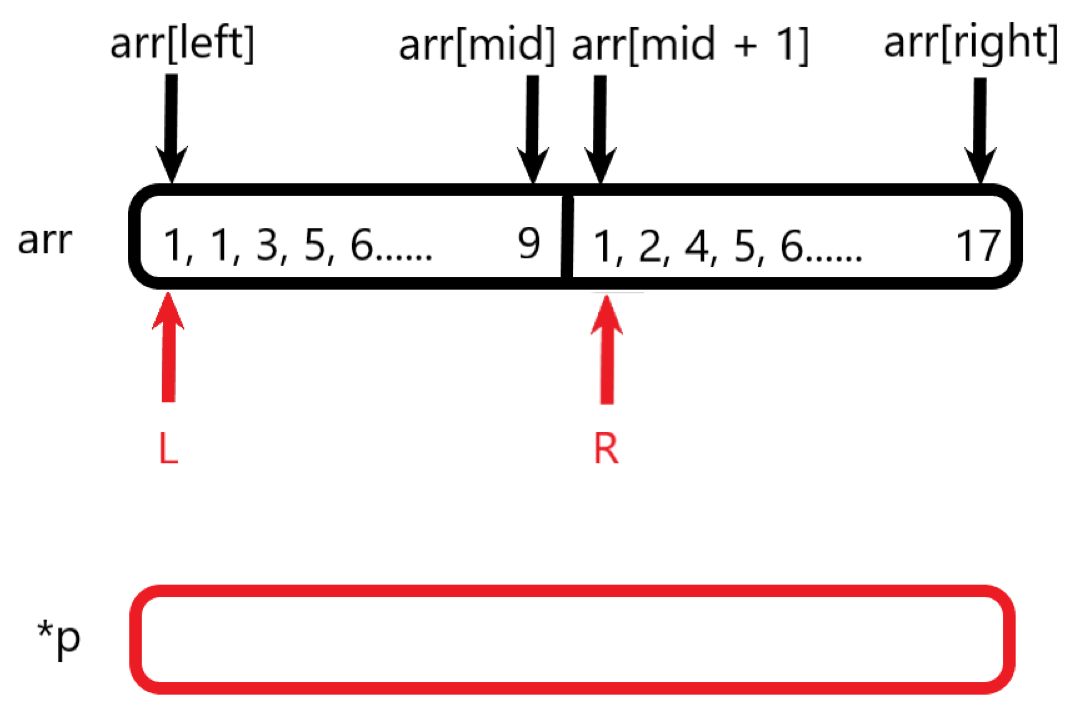2. 第一次比较拷贝：arr[L] 不大于 arr[R]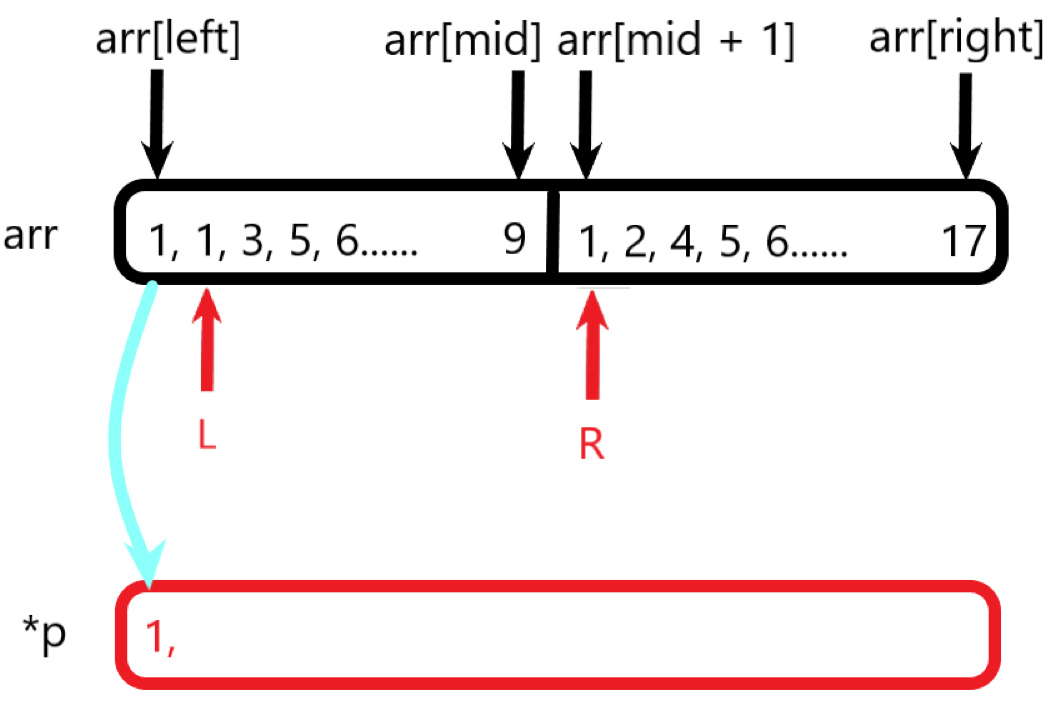3. 第二次: arr[L] 不大于 arr[R]； 第三次 arr[L] 大于 arr[R]；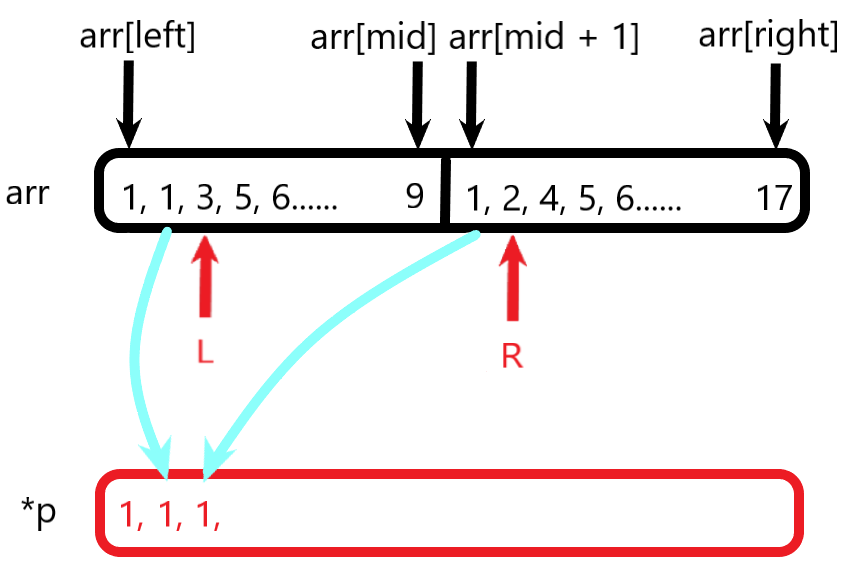4.  第四次：arr[L] 大于 arr[R]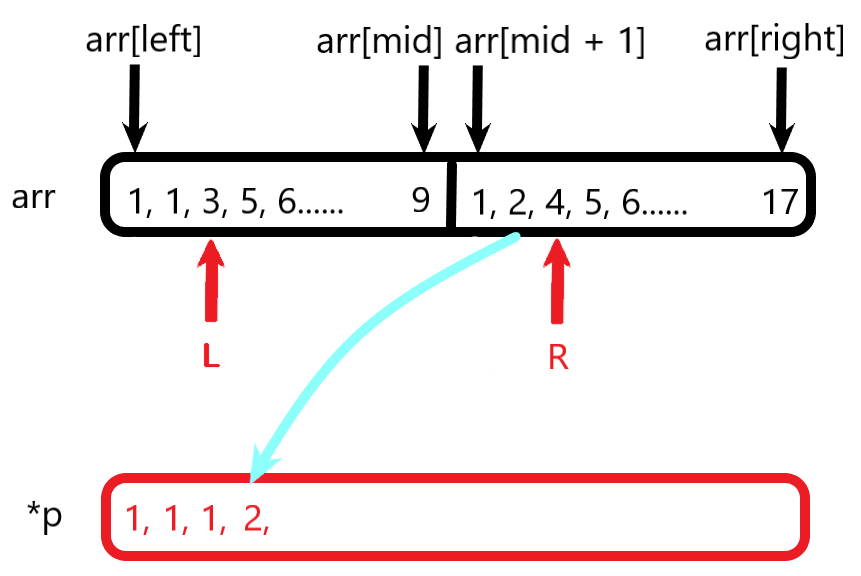5. 第五次：arr[L] 不大于 arr[R]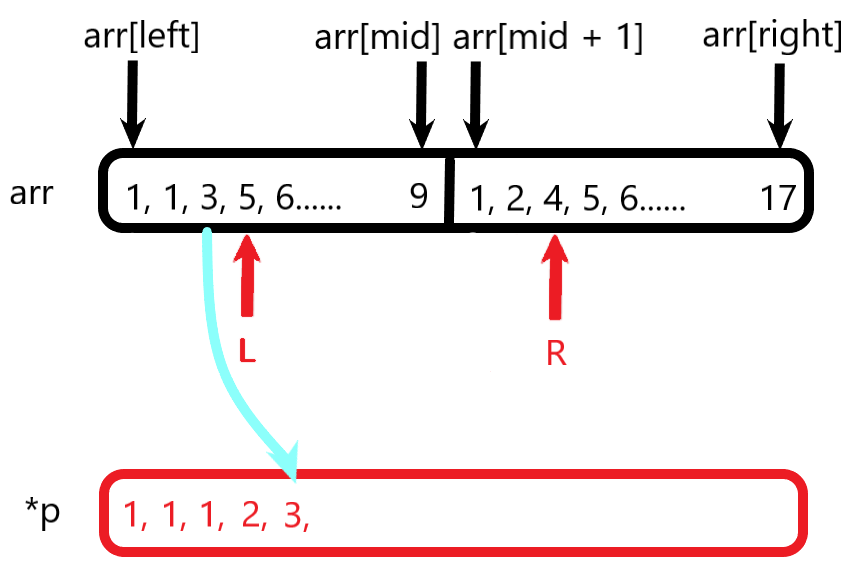6. ……
7. 当左边或者右边遍历完成且全部拷贝，此时指针L越过了mid位到右侧数组，此时结束比较行为，将右侧数组中的剩余数字逐个拷贝进入p中，即指针R之后的数字。如下图所示：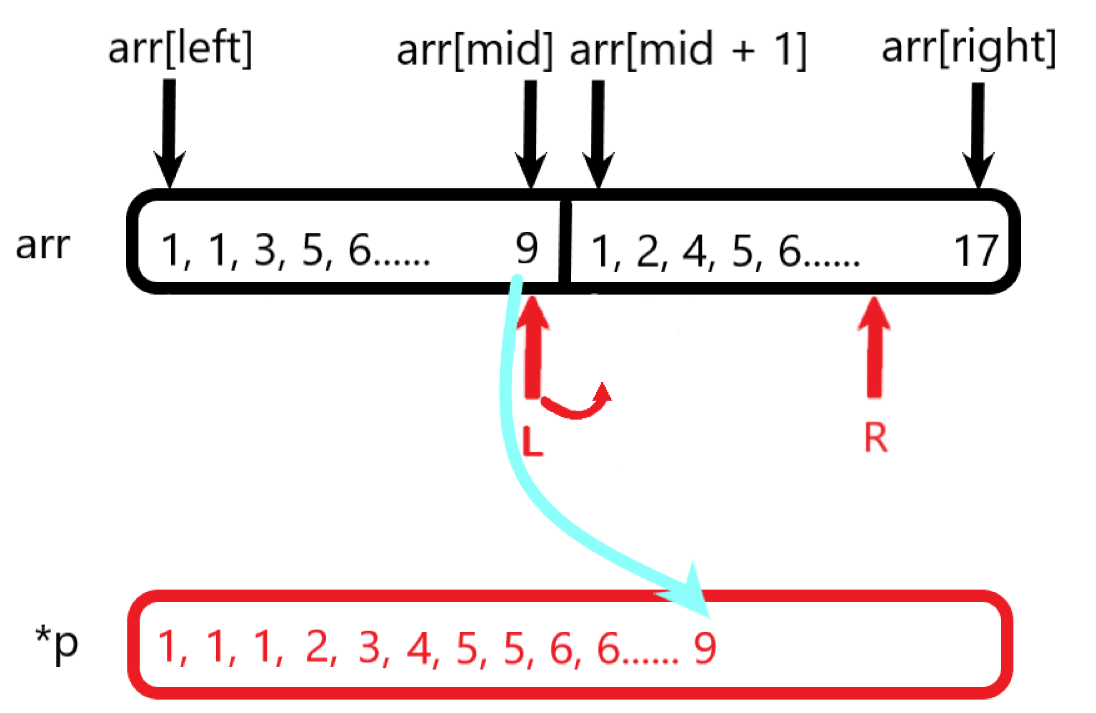8. 将p中的数组拷贝进入原数组arr。
9. 至此从left到right位置的数字就全部拷贝完毕了。

### 递归行为：

每次分别对左侧和右侧进行递归，当left == right结束该次递归。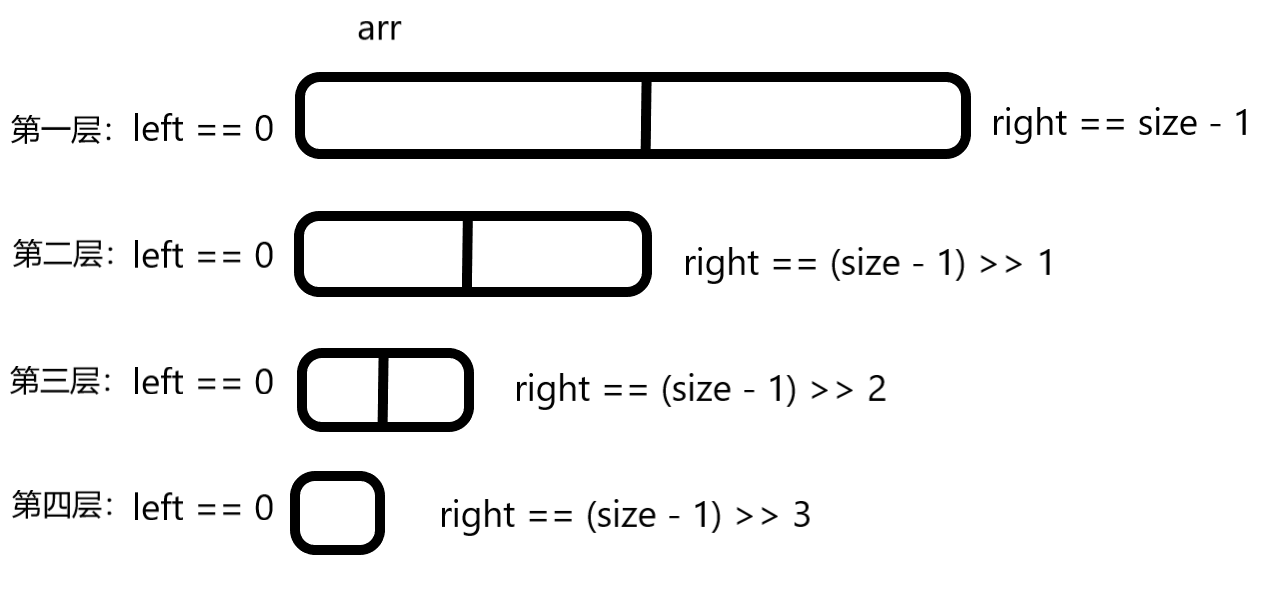left == right时代表left和right指向同一个元素，单个元素必定有序，所以直接返回。

### 代码：

#define _CRT_SECURE_NO_WARNINGS 1
#include <stdio.h>
#include <malloc.h>
#include <windows.h>
#include <memory.h>
//归并排序：
/*
arr:指定数组
left:判定左边界
right:判断右边界
mid:判断中点
*/
void Merge(int* arr, int left, int right, int mid)
{
int *p = (int*)malloc((right - left + 1) * sizeof(int));
int *temp = p;
memset(p, 0, (right - left + 1) * sizeof(int));
int L = left;
int R = mid + 1;
while (L <= mid && R <= right)
{
*p++ = arr[L] < arr[R] ? arr[L++] : arr[R++];
}
while (L <= mid)
{
*p++ = arr[L++];
}
while (R <= right)
{
*p++ = arr[R++];
}

memcpy(arr + left, temp, (right - left + 1) * sizeof(int));

free(temp);
temp = NULL;
}

void MergeSort(int* arr, int left, int right)
{
if (left == right)
return;
int mid = left + ((right - left)>>1);

MergeSort(arr, left, mid);			//左半边
MergeSort(arr, mid + 1, right);		//右半边
Merge(arr, left, right, mid);		//排序
}

int main()
{
int arr = { 9,8,3,5,2,1,6,4,7,10};
MergeSort(arr, 0, sizeof(arr)/ sizeof(arr) - 1);

for (int i = 0; i < sizeof(arr) / sizeof(arr); i++)
{
printf("%d ", arr[i]);
}

system("pause");
return 0;
}

展开全文排序算法
• 主要介绍了C语言 实现归并排序算法的相关资料,需要的朋友可以参考下
• 排序算法中的归并排序(Merge Sort)是利用”归并”技术来进行排序。归并是指将若干个已排序的子文件合并成一个有序的文件。 一、实现原理： 1、算法基本思路 设两个有序的子文件(相当于输入堆)放在同一向量中相邻的...
• 归并排序（Merge sort，台湾译作：合并排序）是建立在归并操作上的一种有效的排序算法。该算法是采用分治法（Divide and Conquer）的一个非常典型的应用。 算法步骤： 1. 申请空间，使其大小为两个已经排序序列之...
• 本文实例为大家分享了C语言实现归并排序的具体代码，供大家参考，具体内容如下 归并排序的基本思想： 将两个及其以上的有序表合并为一张有序表，把待排序序列通过分治法分为若干个有序子序列，然后每两个子序列合并...
• C语言实现归并排序算法归并排序是创建在归并操作上的一种有效的排序算法。下面小编为大家整理了C语言实现归并排序算法，希望能帮到大家！归并排序(Merge sort)是创建在归并操作上的一种有效的排序算法。该算法是采用...

C语言实现归并排序算法

归并排序是创建在归并操作上的一种有效的排序算法。下面小编为大家整理了C语言实现归并排序算法，希望能帮到大家！

归并排序(Merge sort)是创建在归并操作上的一种有效的排序算法。该算法是采用分治法(Divide and Conquer)的一个非常典型的应用。

一个归并排序的例子:对一个随机点的链表进行排序

算法描述

归并操作的.过程如下：

申请空间，使其大小为两个已经排序序列之和，该空间用来存放合并后的序列 设定两个指针，最初位置分别为两个已经排序序列的起始位置 比较两个指针所指向的元素，选择相对小的元素放入到合并空间，并移动指针到下一位置 重复步骤3直到某一指针到达序列尾 将另一序列剩下的所有元素直接复制到合并序列尾

特点：归并排序是稳定的排序.即相等的元素的顺序不会改变, 速度仅次于快速排序，但较稳定。

归并操作

归并操作(merge)，也叫归并算法，指的是将两个顺序序列合并成一个顺序序列的方法。

如：设有数列 [6，202，100，301，38，8，1]

初始状态：6, 202, 100, 301, 38, 8, 1

第一次归并后：[6, 202], [100, 301], [8, 38], ，比较次数：3；

第二次归并后：[6, 100, 202, 301]，[1, 8, 38]，比较次数：4；

第三次归并后：[1, 6, 8, 38, 100, 202, 301]，比较次数：4；

总的比较次数为：3+4+4=11,；

逆序数为14；

算法实现

// Completed on 2014.10.11 17:20// Language: C99 版权所有(C)codingwu (mail: oskernel@126.com) // 博客地址：http://www.cnblogs.com/archimedes/#include#includevoid merge_sort(int *list, const int first, const int last){ int len= last-first+1;  int left_min,left_max; //左半区域边界  int right_min,right_max; //右半区域边界  int index; int i; int *tmp; tmp = (int *)malloc(sizeof(int)*len); if( tmp == NULL || len <= 0 )  return;  for( i = 1; i < len; i *= 2 ) {  for( left_min = 0; left_min < len - i; left_min = right_max)  {   int j;   right_min = left_max = left_min + i;   right_max = left_max + i;   j = left_min;   if ( right_max > len )    right_max = len;   index = 0;   while( left_min < left_max && right_min < right_max )   {    tmp[index++] = (list[left_min] > list[right_min] ? list[right_min++] : list[left_min++]);   }   while( left_min < left_max )   {    list[--right_min] = list[--left_max];   }   while( index > 0 )   {    list[--right_min] = tmp[--index];   }  } } free(tmp);}int main(){ int a[] = {288, 52, 123, 30, 212, 23, 10, 233}; int n, mid; n = sizeof(a) / sizeof(a); mid = n / 2; merge_sort(a, 0, n - 1); for(int k = 0; k < n; k++)  printf("%d ", a[k]); printf("n"); return 0;}

使用递归实现：

// Completed on 2014.10.11 18:20// Language: C99 版权所有(C)codingwu (mail: oskernel@126.com) // 博客地址：http://www.cnblogs.com/archimedes/#include#includevoid merge(int *array,const int first, const int mid, const int last){ int i,index; int first1,last1; int first2,last2; int *tmp; tmp = (int *)malloc((last-first+1)*sizeof(int)); if( tmp == NULL )  return; first1 = first; last1 = mid; first2 = mid+1; last2 = last; index = 0; while( (first1 <= last1) && (first2 <= last2) ) {  if( array[first1] < array[first2] )  {   tmp[index++] = array[first1];   first1++;  }  else{   tmp[index++] = array[first2];   first2++;  } } while( first1 <= last1 ) {  tmp[index++] = array[first1++]; } while( first2 <= last2 ) {  tmp[index++] = array[first2++]; } for( i=0; i

【C语言实现归并排序算法】相关文章：

展开全文• 目录前言：归并排序1.递归版本 演示：实现：2.非递归版本演示：实现： ❥(ゝω・✿ฺ) hi~ 欢迎大家点开我的文章~ 这里我会介绍归并排序的两种实现方法：递归和非递归。

目录

前言：

归并排序

1.递归版本

演示：

实现：

2.非递归版本

演示：

实现：

# 前言：

❥(ゝω・✿ฺ) hi~ 欢迎大家点开我的文章~ 这里我会介绍归并排序的两种实现方法：递归和非递归

注：下面算法我均是使用的升序，降序只需要在执行条件相反就好了~<*))>>=<

# 归并排序

归并排序是建立在归并操作上的一种有效，稳定的排序算法，该算法是采用分治法（Divide and Conquer）的一个非常典型的应用。将已有序的子序列合并，得到完全有序的序列；即先使每个子序列有序，再使子序列段间有序。若将两个有序表合并成一个有序表，称为二路归并

归并：归并实际上就是先将这个整体分成两部分，然后这两部分要是有序，就进行归并操作，结合成一个整体就有序了，如果这两个子部分没有序的就继续分，分到单体或者不存在的时候就有序了，然后再逐步回溯。

分治：“分而治之”，意思就是把一个复杂的问题分成几个小问题，然后再分，分到可以简单求解，然后原问题的解实际上就是这些小问题的解的合并。这里的应用就是将其分成小块进行排序，最后集中到一起在排一次就排序好了。

## 1.递归版本

### 演示：

（下面以2 6 3 0 1 4 5这串待排序数组进行演示）

1.逐步分成可解决问题(单个或者不存在即就是有序的)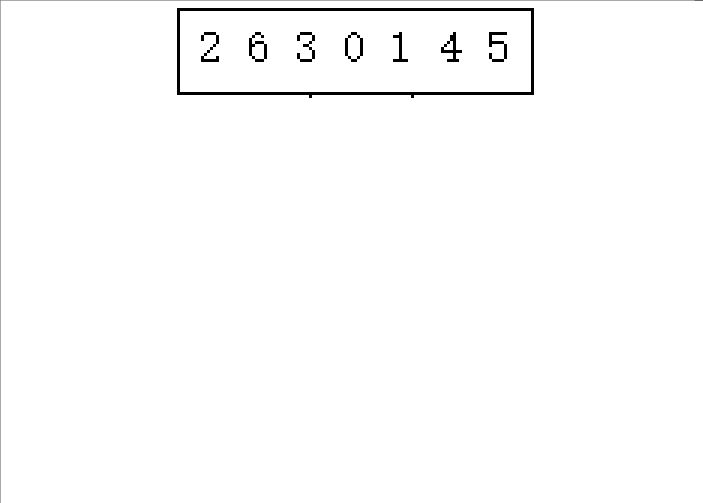2.从后往前归并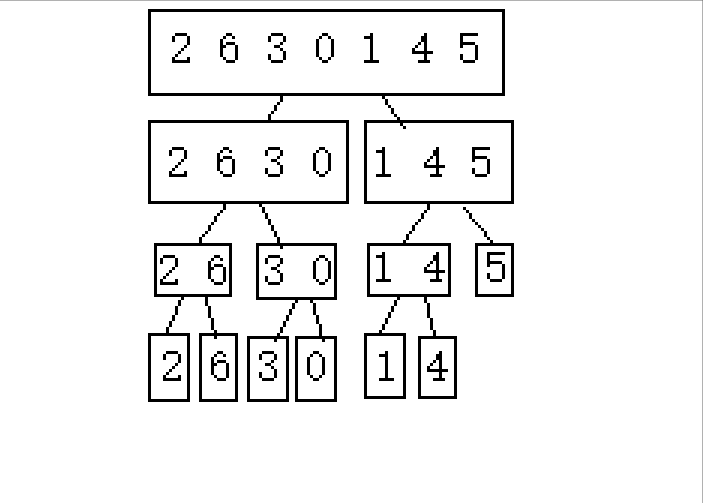### 实现：

经过上面的演示，我们不难发现，首先是要将整个待排序数逐步分成小块，然后进行归并。这明显就有点类似后序算法，即你先把左右两边处理好，然后我在进行我的归并。

基于这一点，程序不难实现：

//辅助归并排序递归的子函数
void _MergeSort(int* a, int* tmp, int begin, int end)
{
if (begin >= end)
return;//单个或者不存在其区间就结束递归

//类似于后序：
int middle = (begin + end) / 2;
int begin1 = begin;
int end1 = middle;
int begin2 = middle + 1;
int end2 = end;
_MergeSort(a, tmp, begin1, end1);
_MergeSort(a, tmp, begin2, end2);

//此时认为 [begin1, end1] 和 [begin2, end2]两段区间上有序
//归并算法
int i = begin;
while (begin1 <= end1 && begin2 <= end2)
{
if (a[begin1] <= a[begin2])
{
tmp[i++] = a[begin1++];
}
else
{
tmp[i++] = a[begin2++];
}
}
while (begin1 <= end1)
{
tmp[i++] = a[begin1++];
}
while (begin2 <= end2)
{
tmp[i++] = a[begin2++];
}
memcpy(a + begin, tmp + begin, sizeof(int) * (end - begin + 1));//内存操作函数，可以将整个数组的数复制过去

}

//归并排序 递归版本
void MergeSort(int* a, int n)
{
//首先malloc一个数组
int* tmp = (int*)malloc(sizeof(int) * n);
if (tmp == NULL)
{
printf("未能申请到内存\n");
exit(-1);
}
//第一次传入 0 和 n - 1 传入闭区间
_MergeSort(a, tmp, 0, n - 1);
free(tmp);
}


tmp数组：我们在归并时，需要一个媒介来进行数字的交换。如果就在原数组上进行操作的话，会覆盖掉原来的数据，造成数据丢失。

mallocvoid *malloc( size_t size ); 向堆内存申请指定字节的连续空间。

memcpy：void* memcpy(void* dest, const void* src, size_t count)；将一段指定字节的连续空间的内容复制到dest。

_MergeSort：用于递归的子函数，在实现归并的前面，首先将两边递归进去，直到发现满足begin >= end的时候就结束递归，回溯上一个递归的函数进行下一步操作，这样就能保证在进行归并前，两个区间均有序。

## 2.非递归版本

递归版本由于数据量过大的话，迭代过多，会导致栈溢出，如果我们能实现非递归版本，使其借用堆内存进行排序，堆内存比栈内存大多了，就不用担心溢出的问题了。

非递归版本实现有点特殊。相信大家也了解过快速排序的非递归版本，那个是利用的栈或者队列数据结构来存储区间从而实现的。但是是否适用于这里的归并排序呢。

通过前面的递归版本我们知道，此归并排序是要在前面排好后也就是后序的形式对这两个区间的数进行归并。如果利用栈来存这些区间，首先存储0 到n - 1，然后存储 0 到middle，middle + 1到 end ，然后就不能直接取出，因为不能确定这两个区间是否是有序的，所以需要继续存进去区间，相对麻烦。

那么我们换个思路，不是所以的非递归实现需要借助类似于栈之类的数据空间的啊，比如斐波那契数列的非递归实现，那么我们直接实现是不是也可以呢？

### 演示：

1.属于2的n次方的排列数([0 7])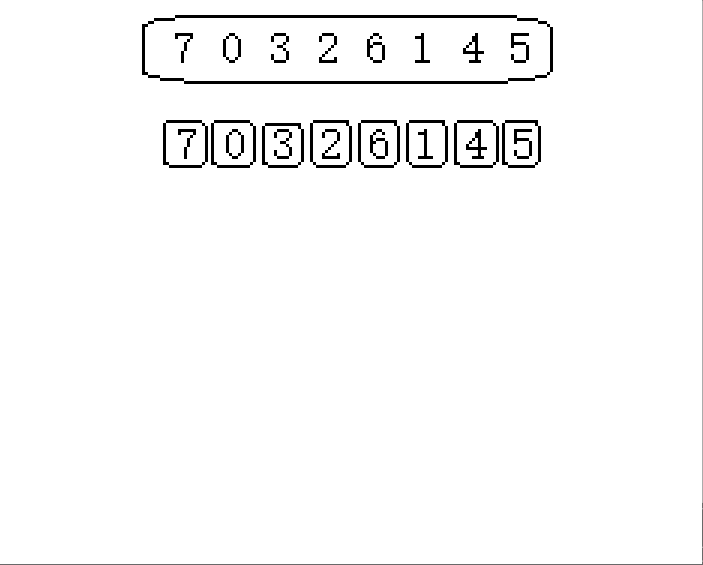注意，在此演示中，每次均是被均分下来的，两份两份。为了实现代码的准确性，那么就要区分 不是2的n次方的奇数次和偶数次。

2.不属于2的n次方的奇数次排列([0 6])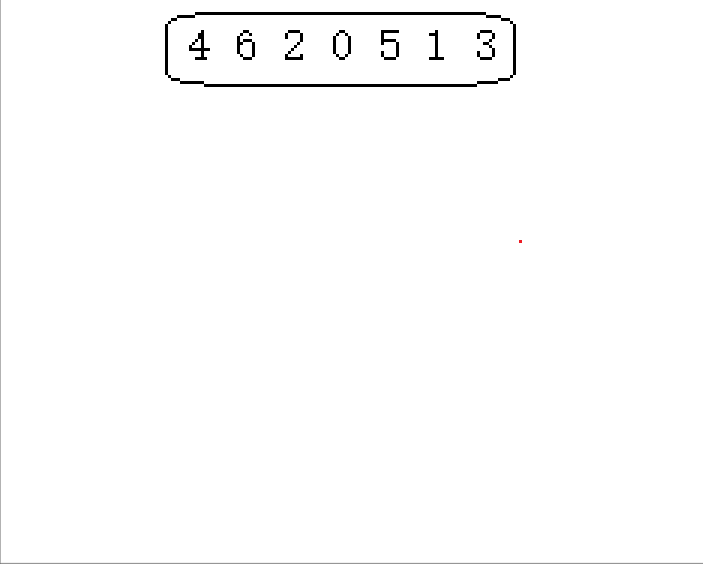这里就会发现，第一次对区间1进行2份2份的分下来的话3没有与其分的，那么3就保持不变，直到有2分分到它这一组的时候就可以正常进行了。

3.不属于2的n次方的偶数次排列([0 9])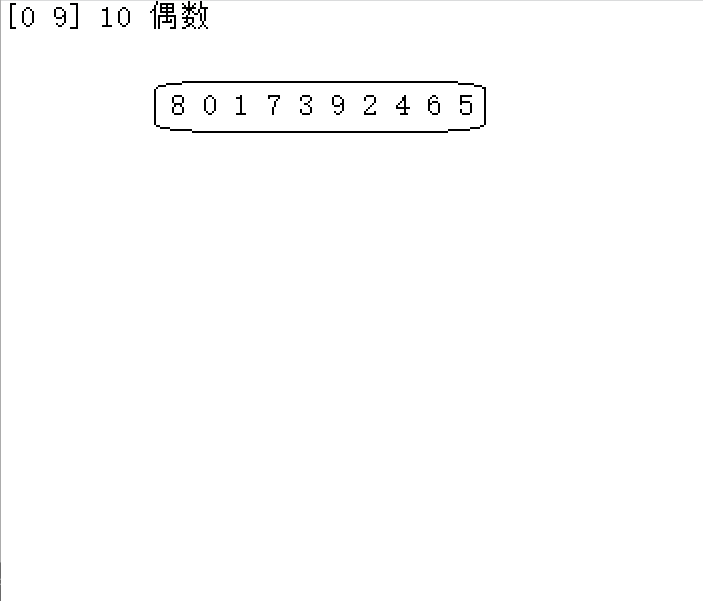这里同样的可以发现，第一次是均可以2分的，但是后面就不行了，所以在后面二分好了后，对应的没有组的也保持不变，直到对应有组。

### 实现：

直接进行分开，然后进行归并。此代码并不好写，主要是区分上面演示中的三种情况。

我们可以定义一个gap变量来确定每一次的区间长度，第一次1，第二次2，第三次4，直到<n的情况，如果待排数组长度不是2的n次方的话，就要对其进行处理。

下面的程序中有两种处理方法，均针对于上述演示中的另外两种情况。

//归并排序 非递归版本
void MergeSortNonR(int* a, int n)
{
int* tmp = (int*)malloc(sizeof(int) * n);
if (tmp == NULL)
{
printf("申请空间失败\n");
exit(-1);
}
//整体交换 - 1
//int gap = 1;
//while (gap < n)
//{
//	for (int i = 0; i < n; i += 2 * gap)//每次跨越两组
//	{
//		int begin1 = i, end1 = i + gap - 1;              // [0, 0] [2, 2] [4, 4] [6, 6]
//		int begin2 = i + gap, end2 = i + 2 * gap - 1;    // [1, 1] [3, 3] [5, 5] [7, 7]

//		//边界调整：begin1肯定不会越界，当end1越界的时候
//		if (end1 >= n)
//		{
//			end1 = n - 1;
//			//让2系列的不存在即可
//			begin2 = 1;
//			end2 = 0;
//		}
//		else if (begin2 >= n)
//		{
//			begin2 = 1;
//			end2 = 0;
//		}
//		else if (end2 >= n)
//		{
//			end2 = n - 1;
//		}
//		//归并
//		int j = begin1;
//		while (begin1 <= end1 && begin2 <= end2)
//		{
//			if (a[begin1] <= a[begin2])
//			{
//				tmp[j++] = a[begin1++];
//			}
//			else
//			{
//				tmp[j++] = a[begin2++];
//			}
//		}
//		while (begin1 <= end1)
//		{
//			tmp[j++] = a[begin1++];
//		}
//		while (begin2 <= end2)
//		{
//			tmp[j++] = a[begin2++];
//		}
//	}
//	memcpy(a, tmp, sizeof(int) * n);//整体复制，那么 不是2的次方倍的n就要进行边界调整
//	gap *= 2;
//}

//每次交换 - 2
int gap = 1;
while (gap < n)
{
for (int i = 0; i < n; i += 2 * gap)//每次跨越两组
{
int begin1 = i, end1 = i + gap - 1;
int begin2 = i + gap, end2 = i + 2 * gap - 1;

if (end1 >= n || begin2 >= n)
{
break;
}
else if (end2 >= n)
{
end2 = n - 1;
}
//归并
int j = begin1;
int m = end2 - begin1 + 1;
while (begin1 <= end1 && begin2 <= end2)
{
if (a[begin1] <= a[begin2])
{
tmp[j++] = a[begin1++];
}
else
{
tmp[j++] = a[begin2++];
}
}
while (begin1 <= end1)
{
tmp[j++] = a[begin1++];
}
while (begin2 <= end2)
{
tmp[j++] = a[begin2++];
}
memcpy(a + i, tmp + i, sizeof(int) * m);//局部复制，end2 - begin1 + 1
}

gap *= 2;
}
free(tmp);

}


gap：gap被定义成每次划分的区间长度，下一次就是上一次两个区间合并一起的区域，所以gap每次循环*2即可。

begin：begin1和2分别是两个区间开始的下标。根据i的区分，i第一次是0，那么根据gap的区域长度，begin1自然是i，begin2就是已经跨过了一个区域了，所以是i+gap

end：end1和2是两个区域结束的下标，end1是第一个区域的最后下标，自然是第二个区域的开始减一，所以是i+gap - 1.end2是第二个区域的最后下标，所以自然是第三个区域的开始减一，即i+gap*2-1。

对于两种特殊情况，有两种处理办法，实际上也就是一种，只不过针对于memcpy是一段一段复制还是整体复制做了区分。一个是不进行处理跳下一步，一个是要进行处理，对边界进行优化即可。

展开全文数据结构 排序算法
• ## C语言——归并排序

千次阅读 2021-12-09 21:56:42
C语言——归并排序 归并排序用到了分治思想，借助递归的方式对一串数字进行排序，整个过程分为分开和合并两个过程。其实归并排序的思想并不难理解，但是用代码实现它却并不容易，我们要写两个函数去分别实现这个过程...排序算法
• C语言实现归并排序 文章目录C语言实现归并排序2路归并排序算法1.定义动态数组2.初始化动态数组3.归并操作4.归并排序算法实现项目完整代码运行效果图 2路归并排序算法 1.定义动态数组 //定义一个动态数组 typedef ...归并排序 2路归并排序
• 归并排序 归并排序将两个有序的序列合并成一个有序的序列。如何得到两个有序的序列，把数据不停的拆分，拆到每个序列只有一个数据。所以归并排序用到了递归思想。 代码 #include <stdio.h> #include <...排序算法 数据结构
• 查找和排序是数据结构...在这个专栏中我们会学习六大查找和十大排序的算法与思想，而本篇将详细讲解其中的归并排序、计数排序、桶排序、基数排序；注意：本文中所有排序按照升序排序，降序只需要把逻辑反过来即可！...排序算法 数据结构
• 主要介绍了C语言演示对归并排序算法的优化实现,归并排序的最差时间复杂度为(nlog n),最优时间复杂为(n),存在可以改进的空间,需要的朋友可以参考下
• ## C语言归并排序算法

千次阅读 2022-02-05 22:17:31
如果说快速排序是让一个数组分成很多小集体进行排序，那么归并排序就是让很多有序的小集体合成一个有序数组。 思路： 如果是升序，对于每一个数字来说其本身是有序的，最初让两个只有一个元素的数组arr1,arr2的...排序算法
• ## 归并排序（递归）——C语言实现

千次阅读 热门讨论 2022-04-11 11:39:43
文章目录一、归并排序定义二、图解归并过程三、动图展示四、分治递归五、归并排序代码 一、归并排序定义 归并排序：是建立在归并操作上的一种有效，稳定的排序算法，该算法是采用分治法（Divide andConquer）的一个...排序算法 数据结构...